• 我们以向量A=[0 1 2 3 4 5 6 7 8] 为例，在MATLAB主窗口中输入如下命令： A = [0 1 2 3 4 5 6 7 8]; find(A) 将会得到 ans = 2 3 4 5 6 7 8 9 （2）返回矩阵中非零元素的位置 find(A) 我们以矩阵A=[1 2 0 1; 0 2 1 ...
（1）返回向量中非零元素的位置 find(A)
我们以向量A=[0 1 2 3 4 5 6 7 8] 为例，在MATLAB主窗口中输入如下命令：
A
= [0 1 2 3 4 5 6 7 8];
find(A)
将会得到
ans =
2 3 4 5 6 7 8 9

（2）返回矩阵中非零元素的位置
find(A)
我们以矩阵A=[1 2 0 1; 0 2 1 1; 0 0 1 1]为例，在matlab中输入find(A)，我们会看到如下结果：
ans
=
1
4
5
8
9
10
11
12
我们可以看到，返回的值并不是我们想象的一个矩阵的形式，仔细观察我们可以看到，如果我们逐列将A的元素进行排序，那么
1 0 0 2 2 0 0 1 1 1 1 1 ，可以看到非零元素位置整好是返回结果的值，一定要注意！所以，当find函数参数为矩阵时，返回的是如上形式的结果！

（3）返回满足某条件的值find(A**)
我们以向量A=[0 1 2 3 4 5 6 7 8] 为例，在MATLAB主窗口中输入，find(A>4),回车得到
ans
=
6 7 8 9
可以看到返回的是A中大于4的元素的位置。

（4）返回前N个非零元素的位置,find(A,X)
X必须为正整数，我们以A=[0
0 2 3 4 5 6 7 8]为例，在MTALAB主窗口中输入如上向量，然后输入find(A,1)，回车，返回第一个非零值的位置，然后继续输入find(A,3)，返回前三个非零值的位置
find(A,1)
ans
=
3
find(A,3)
ans
=
3 4 5
结果正如上面所描述，分别返回第一个非零值的位置3，以及前三个非零值的位置3,4,5

（5）返回最后一个非零值的位置find(A,1,,'last')
我们还是以A=[0 0 2 3 4 5 6 7 8]为例，在MATLAB主窗口中输入find(A,1,'last')，回车
ans
=
9
可以看到返回的是最后一个非零值的位置9

（6）返回最后一个非零值的行列位置
我们还是以A=[0 0 2 3 4 5 6 7 8]为例，在MATLAB主窗口中输入[a,b]=find(A,1,'last')，回车
a =
1
b =
9
可以看到返回的是最后一个非零元素所在的行第一行，列第九列。

（7）[a1,a2] = find(a)，找出a中非零元素所在的行和列，并分别存储在a1和a2中

（8）[a1,a2,v] = find(a)，找出a中非零元素所在的行和列，分别存储在a1和a2中，并将结果放在v中
————————————————
版权声明：本文为CSDN博主「小白的进阶」的原创文章，遵循 CC 4.0 BY-SA 版权协议，转载请附上原文出处链接及本声明。
原文链接：https://blog.csdn.net/laobai1015/article/details/58058017

展开全文• ## MATLABfind函数用法

千次阅读 2018-06-17 19:36:10
ind = find(X) ind = 1 3 4 8 9返回的是向量X中的非零元素索引值2，X = [1 0 4 -3 0 0 0 8 6]; ind = find(X&gt;2) ind = 3 8 9返回向量中满足大于2的元素的索引值3，当X是个矩阵时，X = ...
1，X = [1 0 4 -3 0 0 0 8 6];
ind = find(X)

ind =

1     3     4     8     9返回的是向量X中的非零元素索引值2，X = [1 0 4 -3 0 0 0 8 6];
ind = find(X>2)

ind =

3     8     9返回向量中满足大于2的元素的索引值3，当X是个矩阵时，X = [3 2 0; -5 0 7; 0 0 1];

[r,c,v] = find(X>2)

r =

1
2

c =

1
3

v =

1
1r表示行，c表示列，v为1表示为真。此处表示第一行第一列和第二行第三列符合要求（>2）;
展开全文• find函数：用于查询非零元素的行和列标志 语法： ind = find(X) ind = find(X, k) ind = find(X, k, 'first') ind = find(X, k, 'last') [row,col] = find(X, ...) [row,col,v] = find(X...
find函数：用于查询非零元素的行和列标志语法：

ind = find(X)
ind = find(X, k)
ind = find(X, k, 'first')
ind = find(X, k, 'last')
[row,col] = find(X, ...)
[row,col,v] = find(X, ...)
复制代码
ind = find(X)  查询非零元素的位置，如果X是一个行向量，则返回一个行向量，否则，返回一个列向量。如果X全是零元素或者是空数组，则返回一个空数组。[row,col V] = find(X, ...)  查询满足一定条件的元素的行和列X一般为逻辑表达式，如X>5等。其中   row 返回满足条件的元素行的位置       col 返回满足条件的元素的列的位置       V   如果X是一个逻辑表达式，则返回一个逻辑数组，一般如果存在满足要求的元素，则返回一个单位列向量。如果不存在满足要求的元素，则返回一个空向量。例题：

>> A= magic(4)

A =

16     2     3    13
5    11    10     8
9     7     6    12
4    14    15     1
复制代码

>> [r,c,v]= find(A>12)

r =

1
4
4
1

c =

1
2
3
4

v =

1
1
1
1
复制代码
例题：

>> x = [3 0 0; 0 0 7; 0 0 1]

x =

3     0     0
0     0     7
0     0     1

>> [r,c,v]=find(x)

r =

1
2
3

c =

1
3
3

v =

3
7
1
复制代码

>> x = [3 2 0; -5 0 7; 0 0 1];
>> [~,~,V]=find(x~=0)

V =

1
1
1
1
1

>> [~,~,V]=find(x)

V =

3
-5
2
7
1
复制代码
比较两种调用方式返回的V的区别：[r,c,v]= find(A>12)：V返回一个单位列向量[r,c,v]=find(x)    ：V返回由满足要求的元素构成一个列向量ind = find(X, k, 'first')ind = find(X, k, 'last')返回K个满足条件X的元素的行和列的位置，其中'first'表示从第一个开始算起，'last'表示从最后一个元素开始算起。例题

>> a=[4 0 3 1 0 9 2];
>> find(a,3,'first')

ans =

1     3     4

>> find(a,3,'last')

ans =

4     6     7复制代码

复制代码
复制代码

展开全文• 三维曲线plot3函数与plot函数用法十分相似，其调用格式为：plot3(x1,y1,z1,选项1,x2,y2,z2,选项2,…,xn,yn,zn,选项n)其中每一组x,y,z组成一组曲线的坐标参数，选项的定义和plot函数相同。当x,y,z是同维向量时，则x,y...
三维曲线plot3函数与plot函数用法十分相似，其调用格式为：plot3(x1,y1,z1,选项1,x2,y2,z2,选项2,…,xn,yn,zn,选项n)其中每一组x,y,z组成一组曲线的坐标参数，选项的定义和plot函数相同。当x,y,z是同维向量时，则x,y,z 对应元素构成一条三维曲线。当x,y,z是同维矩阵时，则以x,y,z对应列元素绘制三维曲线，曲线条数等于矩阵列数。 例 绘制三维曲线。程序如下：t=0:pi/100:20*pi;x=sin(t);y=cos(t);z=t.*sin(t).*cos(t);plot3(x,y,z);title('Line in 3-D Space');xlabel('X');ylabel('Y');zlabel('Z');三维曲面1．产生三维数据在MATLAB中，利用meshgrid函数产生平面区域内的网格坐标矩阵。其格式为：x=a:d1:b; y=c:d2:d;[X,Y]=meshgrid(x,y);语句执行后，矩阵X的每一行都是向量x，行数等于向量y的元素的个数，矩阵Y的每一列都是向量y，列数等于向量x的元素的个数。2．绘制三维曲面的函数surf函数和mesh函数的调用格式为：mesh(x,y,z,c)：画网格曲面，将数据点在空间中描出,并连成网格。 surf(x,y,z,c)：画完整曲面，将数据点所表示曲面画出。一般情况下，x,y,z是维数相同的矩阵。x,y是网格坐标矩阵，z是网格点上的高度矩阵，c用于指定在不同高度下的颜色范围。 例 绘制三维曲面图z=sin(x+sin(y))-x/10。程序如下： [x,y]=meshgrid(0:0.25:4*pi); %在[0,4pi]×[0,4pi]区域生成网格坐标 z=sin(x+sin(y))-x/10; mesh(x,y,z); axis([0 4*pi 0 4*pi -2.5 1]);此外，还有带等高线的三维网格曲面函数meshc和带底座的三维网格曲面函数meshz。其用法与mesh类似，不同的是meshc还在xy平面上绘制曲面在z轴方向的等高线，meshz还在xy平面上绘制曲面的底座。 例 在xy平面内选择区域[-8,8]×[-8,8]，绘制4种三维曲面图。程序如下：[x,y]=meshgrid(-8:0.5:8);z=sin(sqrt(x.^2+y.^2))./sqrt(x.^2+y.^2+eps);subplot(2,2,1);mesh(x,y,z);title('mesh(x,y,z)')subplot(2,2,2);meshc(x,y,z);title('meshc(x,y,z)')subplot(2,2,3);meshz(x,y,z)title('meshz(x,y,z)')subplot(2,2,4);surf(x,y,z);title('surf(x,y,z)')3．标准三维曲面sphere函数的调用格式为：(球体，领域，半球，圈子)[x,y,z]=sphere(n)：generates three(N+1)-by-(N+1) matrices so that SURF(X,Y,Z) produces a unit sphere.cylinder函数的调用格式为：(圆筒，圆柱体，柱面)[x,y,z]= cylinder(R,n)，其中r为圆周半径，n为组成圆周的点数。MATLAB还有一个peaks 函数，称为多峰函数，常用于三维曲面的演示。 例 绘制标准三维曲面图形。程序如下：t=0:pi/20:2*pi;[x,y,z]= cylinder(2+sin(t),30);subplot(2,2,1);surf(x,y,z);subplot(2,2,2);[x,y,z]=sphere;surf(x,y,z);subplot(2,1,2);[x,y,z]=peaks(30);surf(x,y,z); 其他三维图形在介绍二维图形时，曾提到条形图、杆图、饼图和填充图等特殊图形，它们还可以以三维形式出现，使用的函数分别是bar3、stem3、pie3 和fill3。bar3函数绘制三维条形图，常用格式为：bar3(y)bar3(x,y)stem3函数绘制离散序列数据的三维杆图，常用格式为：stem3(z)stem3(x,y,z)pie3函数绘制三维饼图，常用格式为：pie3(x)fill3函数等效于三维函数fill，可在三维空间内绘制出填充过的多边形，常用格式为：fill3(x,y,z,c) 例 绘制三维图形：(1) 绘制魔方阵的三维条形图。(2) 以三维杆图形式绘制曲线y=2sin(x)。(3) 已知x=[2347,1827,2043,3025]，绘制饼图。(4) 用随机的顶点坐标值画出五个黄色三角形。程序如下：subplot(2,2,1);bar3(magic(4))subplot(2,2,2);y=2*sin(0:pi/10:2*pi);stem3(y);subplot(2,2,3);pie3([2347,1827,2043,3025]);subplot(2,2,4);fill3(rand(3,5),rand(3,5),rand(3,5), 'y' ) 例 绘制多峰函数的瀑布图和等高线图。程序如下：subplot(1,2,1);[X,Y,Z]=peaks(30);waterfall(X,Y,Z)xlabel('X-axis'),ylabel('Y-axis'),zlabel('Z-axis');subplot(1,2,2);contour3(X,Y,Z,12,'k'); %其中12代表高度的等级数xlabel('X-axis'),ylabel('Y-axis'),zlabel('Z-axis');图形修饰处理视点处理MATLAB提供了设置视点的函数view，其调用格式为：view(az,el)其中az为方位角，el为仰角，它们均以度为单位。系统缺省的视点定义为方位角-37.5°,仰角30°。例 从不同视点绘制多峰函数曲面。 程序如下： subplot(2,2,1);mesh(peaks); view(-37.5,30); %指定子图1的视点 title('azimuth=-37.5,elevation=30') subplot(2,2,2);mesh(peaks); view(0,90); %指定子图2的视点 title('azimuth=0,elevation=90') subplot(2,2,3);mesh(peaks); view(90,0); %指定子图3的视点 title('azimuth=90,elevation=0') subplot(2,2,4);mesh(peaks); view(-7,-10); %指定子图4的视点 title('azimuth=-7,elevation=-10')色彩处理1．颜色的向量表示MATLAB除用字符表示颜色外，还可以用含有3个元素的向量表示颜色。向量元素在[0,1]范围取值，3个元素分别表示红、绿、蓝3种颜色的相对亮度，称为RGB三元组。2．色图色图(Color map)是MATLAB系统引入的概念。在MATLAB中，每个图形窗口只能有一个色图。色图是m×3 的数值矩阵，它的每一行是RGB三元组。色图矩阵可以人为地生成，也可以调用MATLAB提供的函数来定义色图矩阵。3．三维表面图形的着色三维表面图实际上就是在网格图的每一个网格片上涂上颜色。surf函数用缺省的着色方式对网格片着色。除此之外，还可以用shading命令来改变着色方式。shadingfaceted命令将每个网格片用其高度对应的颜色进行着色，但网格线仍保留着，其shading flat命令将每个网格片用同一个颜色进行着色，且网格线也用相应的颜色，从而使得图形表面显得更加光滑。shading interp命令在网格片内采用颜色插值处理，得出的表面图显得最光滑。颜色是黑色。这是系统的缺省着色方式 例 3种图形着色方式的效果展示。程序如下：[x,y,z]=sphere(20);colormap(copper);subplot(1,3,1);surf(x,y,z);axis equalsubplot(1,3,2);surf(x,y,z);shading flat;axis equalsubplot(1,3,3);surf(x,y,z);shading interp;axis equal 光照处理MATLAB提供了灯光设置的函数，其调用格式为：light('Color',选项1,'Style',选项2,'Position',选项3) 光照处理后的球面。程序如下：[x,y,z]=sphere(20);subplot(1,2,1);surf(x,y,z);axis equal;light('Posi',[0,1,1]);shading interp;hold on;plot3(0,1,1,'p');text(0,1,1,' light');subplot(1,2,2);surf(x,y,z);axis equal;light('Posi',[1,0,1]);shading interp;hold on;plot3(1,0,1,'p');text(1,0,1,' light'); 图形的裁剪处理例4-22 绘制三维曲面图，并进行插值着色处理，裁掉图中x和y都小于0部分。程序如下：[x,y]=meshgrid(-5:0.1:5);z=cos(x).*cos(y).*exp(-sqrt(x.^2+y.^2)/4);surf(x,y,z);shading interp;pause %程序暂停i=find(x<=0&y<=0);z1=z;z1(i)=NaN;surf(x,y,z1);shading interp;为了展示裁剪效果，第一个曲面绘制完成后暂停，然后显示裁剪后的曲面。 图像处理与动画制作图像处理1．imread和imwrite函数imread和imwrite函数分别用于将图像文件读入MATLAB工作空间，以及将图像数据和色图数据一起写入一定格式的图像文件。MATLAB支持多种图像文件格式，如.bmp、.jpg、.jpeg、.tif等。2．image和imagesc函数这两个函数用于图像显示。为了保证图像的显示效果，一般还应使用colormap函数设置图像色图。例5-23 有一图像文件flower.jpg，在图形窗口显示该图像。程序如下：[x,cmap]=imread('flower.jpg'); %读取图像的数据阵和色图阵image(x);colormap(cmap);axis image off %保持宽高比并取消坐标轴动画制作MATLAB提供getframe、moviein和movie函数进行动画制作。1．getframe函数getframe函数可截取一幅画面信息(称为动画中的一帧)，一幅画面信息形成一个很大的列向量。显然，保存n幅图面就需一个大矩阵。2．moviein函数moviein(n)函数用来建立一个足够大的n列矩阵。该矩阵用来保存n幅画面的数据，以备播放。之所以要事先建立一个大矩阵，是为了提高程序运行速度。3．movie函数movie(m,n)函数播放由矩阵m所定义的画面n次，缺省时播放一次。例 绘制了peaks函数曲面并且将它绕z轴旋转。程序如下[X,Y,Z]=peaks(30);surf(X,Y,Z)axis([-3,3,-3,3,-10,10])axis off;shading interp;colormap(hot);m=moviein(20); %建立一个20列大矩阵for i=1:20view(-37.5+24*(i-1),30) %改变视点m(:,i)=getframe; %将图形保存到m矩阵endmovie(m,2); %播放画面2次MATLAB三维网图的高级处理4.1. 消隐处理例.比较网图消隐前后的图形z=peaks(50);Subplot(2,1,1);mesh(z);title('消隐前的图')hidden offsubplot(2,1,2);mesh(z);title('消隐后的图')hidden oncolormap([0 0 1])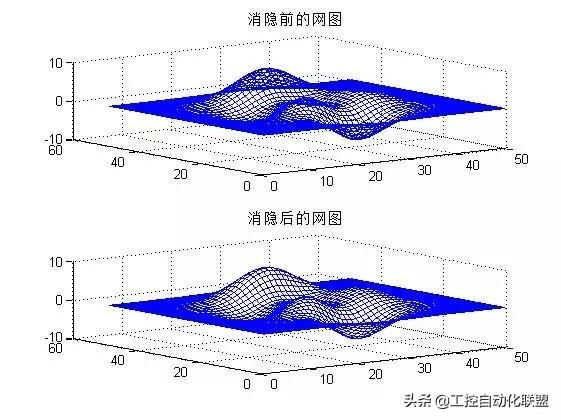4.2. 裁剪处理利用不定数NaN的特点,可以对网图进行裁剪处理例.图形裁剪处理P=peaks(30);subplot(2,1,1);mesh(P);title('裁剪前的图');subplot(2,1,2);P(20:23,9:15)=NaN*ones(4,7); %裁剪meshz(P); %垂帘网线图title('裁剪后的图')colormap([0 0 1]) %蓝色网线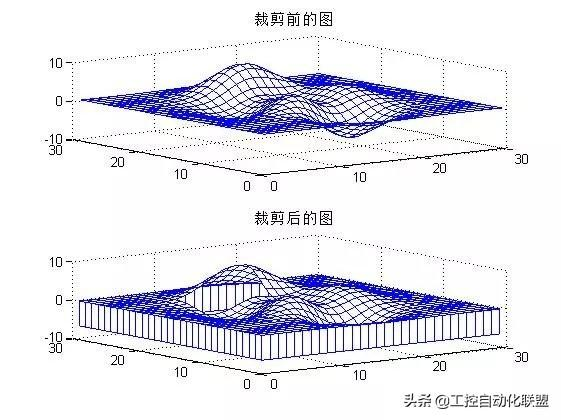注意裁剪时矩阵的对应关系,即大小一定要相同4.3. 三维旋转体的绘制为了一些专业用户可以更方便地绘制出三维旋转体,MATLAB专门提供了2个函数:柱面函数cylinder和球面函数sphere柱面图绘制由函数cylinder实现.[X,Y,Z]=cylinder(R,N)　此函数以母线向量R生成单位柱面.母线向量R是在单位高度里等分刻度上定义的半径向量.N为旋转圆周上的分格线的条数.可以用surf(X,Y,Z)来表示此柱面.[X,Y,Z]=cylinder(R)或[X,Y,Z]=cylinder此形式为默认N=20且R=[1 1]例.柱面函数演示举例x=0:pi/20:pi*3;r=5+cos(x);[a,b,c]=cylinder(r,30);mesh(a,b,c)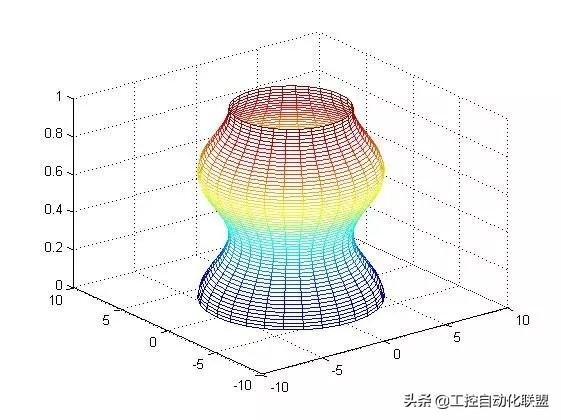例.旋转柱面图.t=0:pi/12:3*pi;r=abs(exp(-0.25*t).*sin(t));r=abs(exp(-0.25*t).*sin(t));[X,Y,Z]=cylinder(r,30);mesh(X,Y,Z)colormap([1 0 0])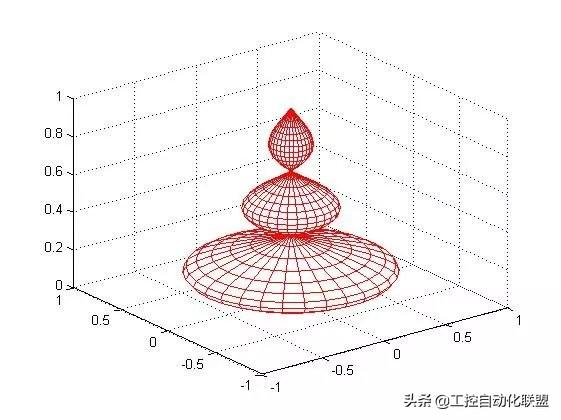(2).球面图球面图绘制由函数sphere来实现[X,Y,Z]=sphere(N)%此函数生成3个(N+1)*(N+1)的矩阵surf(X,Y,Z) %产生单位球面[X,Y,Z]=sphere %此型式使用了默认值N=20.Sphere(N) %只是绘制了球面图而不返回任何值例.绘制地球表面的气温分布示意图.[a,b,c]=sphere(40);t=abs(c);surf(a,b,c,t);axis('equal') %此两句控制坐标轴的大小相同axis('square')colormap('hot')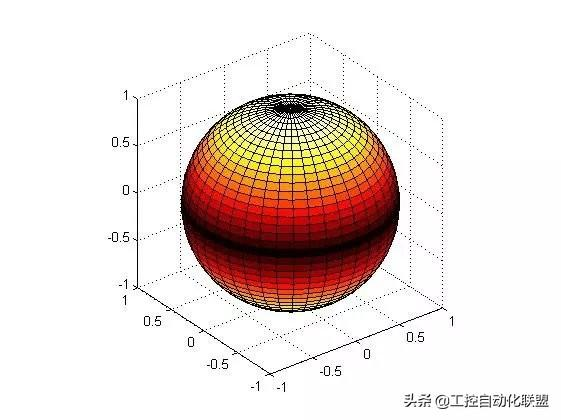展开全文• 有那些相见恨晚的MATLAB绘图命令MATLAB的绘图功能可谓非常的强大，常用的有plot,plot3,subplot,scatter等等，这些函数有许多的参数设置，可以画出各种需求的图。但是这些强大的绘图命令也有无能为力的时候。为什么呢...
• ind = find(X) 查询非零元素的位置 如果X是一个行向量，则返回一个行向量， 否则，返回一个列向量。 如果X全是零元素或者是空数组，则返回一个空数组。 [row,col V] = find(X, ...) 查询...
• ## matlab中find函数用法

万次阅读 多人点赞 2019-03-06 15:10:09
1.返回素有非零元素的位置 例如： 注：竖着数！！ 2.条件：find（A==1） 例如：返回的仍然是位置！ 3.返回前N个非零元素的位置,find(A,X...6.[a,b,v] = find(A)，找出A中非零元素所在的行和列，分别存储在a和b中，...
• ## Matlab中find函数用法

千次阅读 2014-08-05 08:57:02
• matlabfind() 函数用法 I.用法一: b=find(a),a是一个矩阵,查询非零元素的位置，如果X是一个行向量，则返回一个行向量;否则，返回一个列向量。如果X全是零元素或者是空数组，则返回一个空数组,例子如下所示,也...
• find()函数是用来查询满足要求非零值在矩阵中位置的函数，可以返回元素在其中的位置等，下面就通过实例介绍一下不同函数用法。 返回向量中非零元素的位置 find(A) 我们以向量A=[0 1 2 3 4 5 6 7 8] 为例...学习 转载
• ## MATLAB中find函数用法

千次阅读 2015-09-12 21:03:43
find()函数是用来查询满足要求非零值在矩阵中位置的函数，可以返回元素在其中的位置等，下面就通过实例介绍一下不同函数用法。 返回向量中非零元素的位置 find(A) 我们以向量A=[0 1 2 3 4 5 6 7 8] 为例...
• matlab find函数婚姻是不可捉摸在于：一个乐观的男人加上一个乐观的女人，有时等于两个悲观主义者。x1=-2:2; k1=-2:2; x2=[1,-1,1]; k2=-1:1; k=min([k1,k2]):max([k1,k2])举个例子讲下find函数用法： 例如A=[1 2 ...
• MATLABfind函数用法 find函数：查找非零元素的索引和值 主要有下面5中用法， k = find(X) X有三种情况： （1）：如果X为向量（行向量或者列向量），k为X中非零元素索引组成的向量。k的方向与X的方向相同。如果X...
• ## Matlabfind()函数用法总结

万次阅读 多人点赞 2017-02-27 13:34:33
1 2 3 4 5 6 7 8] 为例，在MATLAB主窗口中输入如下命令： A = [0 1 2 3 4 5 6 7 8]; find(A) 将会得到 ans =  2 3 4 5 6 7 8 9 （2）返回矩阵中非零元素的位置 find(A) 我们以矩阵A=[1 2 0 1; ...
• ## Matlabfind函数

千次阅读 2015-02-04 22:45:54
MATLAB中矩阵元素的查找函数find的一些基本使用，简单的例子说明函数的主要用法。欢迎MATLAB的初学者一起学习讨论。查找
• ## matlabfind函数详解

万次阅读 2018-07-16 23:05:40
Find 这个函数用处也挺大的，这几天看很多程序都见到这一函数，今天要好好给阐述，了解下 这个函数是为了找到矩阵或者是数组，向量中的非零元素。下面一大段英文没耐心看。看看例子就行了。 第一个用法是 ndices = ...
• find   找到非零元素的索引和值     语法： 1. ind = find(X) 2. ind = find(X, k) 3. ind = find(X, k, 'first') 4. ind = find(X, k, 'last') 5. [row,col] = find(X, ...) 6. [row,col,v...
• http://jingyan.baidu.com/album/3c343ff71444890d3679635d.html?picindex=1
• 基本用法 返回矩阵或向量中非零元素的索引 注意：matlab中下标从1开始 举例： （1）向量 返回非零元素下标 find（vector） x=[1 2 3 0 0 6 7 8 9]; find(x) ans = 1 2 3 6 7 8 9 返回前k个非零元素的下标 ...
• Matlabfind()函数用法总结 其主要功能为返回某类元素在向量或者矩阵中的位置。 1、返回向量中非零元素的位置 示例：find(A) 2、返回矩阵中非零元素的位置 示例：find(A) 3、返回满足某条件值的位置 示例：find(A&...
• a=[0 0 0 0 0 0 0 1 0 0 0 1 1 1 0 0 0 1 0 0 0 0 0 0 0] a=double(a); [i,j]=find(a>0.5);# matlabfind函数用法matlab 订阅